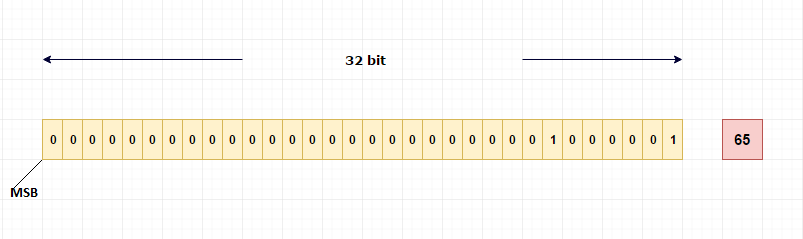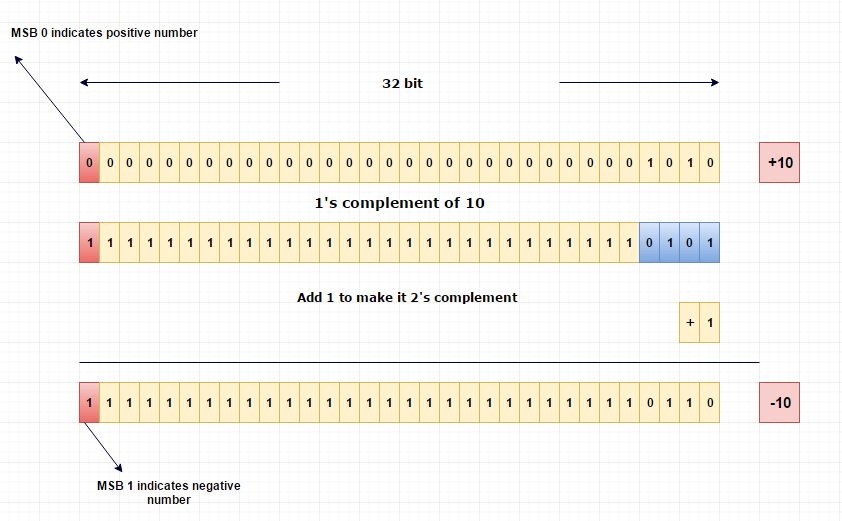# How integers are stored in memory?

Integers are whole numbers which will be stored in computer using 4 bytes (32 bit) of memory.

#### Example

65

Binary equivalent of 65 is (1000001) 2 .

The MSB (most significant bit) bit is used to indicate whether the number is positive or negative.

For positive numbers MSB will be 0.

For negative numbers MSB will be 1.

In our case, 65 is positive so MSB will be 0.

This binary equivalent of 65 will be stored in 32-bit memory like below,

#### Pictorial Explanation# How negative integers are stored in memory?

Computer uses special mechanism to store negative numbers which is 2’s complement format.

Before going to that, let’s have a look on 1’s complement of a number.

#### 1’s complement of a number

1’s compliment of number is just inverting binary bits of an actual number.

Example:

10

Binary representation will be (1010) 2

1’s compliment of 10 is, 0101 (switching 0 to 1 and 1 to 0).

#### 2’s complement of a number

To get 2’s complement of a number, just add 1 to 1’s complement of an actual number.

#### Pictorial ExplanationThis 2’s complement form will be stored in computer.

## Useful Resources

https://en.wikipedia.org/wiki/Ones%27_complement
https://en.wikipedia.org/wiki/Two%27s_complement